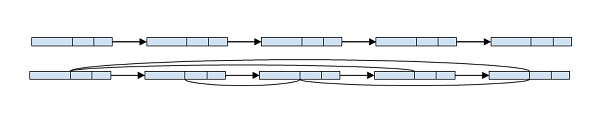# Point to next higher value node in a linked list with an arbitrary pointer in C++

In this problem, we are given a linked list with a value, link pointer and an arbitrary pointer. Our task is to make the arbitrary pointer point to point the next large value in the list.

Let’s take an example to understand the problem,Here, we can see 8 points to 12, 12 to 41, 41 to 54, 54 to 76 which are successive larger elements of the linked list.

To solve this problem, we will use the merge sort algorithm to sort elements and use the sort as a linked list for the arbitrary pointer.

For this we will use the merge sort algorithm on linked list treating the arbitrary pointer as a primary pointer for sorting and linked list, this will solve the problem i.e. each arbitrary point will point to the next larger node then it.

## Example

Program to show an implementation of our solution,

Live Demo

#include <iostream>
using namespace std;
class Node {
public:
int data;
Node* next, *arbit;
};
Node* SortedMerge(Node* a, Node* b);
void FrontBackSplit(Node* source, Node** frontRef, Node** backRef);
Node* a, *b;
return;
MergeSort(&a);
MergeSort(&b);
}
Node* SortedMerge(Node* a, Node* b) {
Node* result = NULL;
if (a == NULL)
return (b);
else if (b == NULL)
return (a);
if (a->data <= b->data){
result = a;
result->arbit = SortedMerge(a->arbit, b);
} else {
result = b;
result->arbit = SortedMerge(a, b->arbit);
}
return (result);
}
void FrontBackSplit(Node* source, Node** frontRef, Node** backRef) {
Node* fast, *slow;
if (source == NULL || source->arbit == NULL){
*frontRef = source;
*backRef = NULL;
return;
}
slow = source, fast = source->arbit;
while (fast != NULL){
fast = fast->arbit;
if (fast != NULL){
slow = slow->arbit;
fast = fast->arbit;
}
}
*frontRef = source;
*backRef = slow->arbit;
slow->arbit = NULL;
}
Node* new_node = new Node();
new_node->data = new_data;
new_node->arbit = NULL;
}
while (temp != NULL){
temp->arbit = temp->next;
temp = temp->next;
}
}
int main() {
cout << "\t\tArbitrary pointer overlaoded \n Traversing linked List\n";
cout<<"Using Next Pointer\n";
cout << head->data << ", ";
}
printf("\nUsing Arbit Pointer\n");
}
return 0;
}

## Output

Arbitrary pointer overlaoded
12, 32, 45, 87,